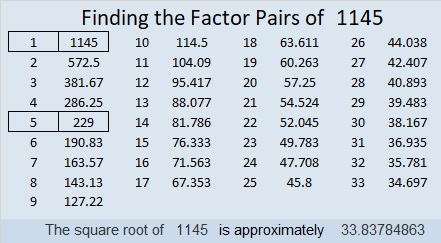# 1145 Mysterious Butterfly

This adventurous butterfly invites you to solve its mystery. Be warned, it might not be as easy as it seems. Don’t write any factors down unless you are SURE they belong where you’re putting them. Let logic be your guide.Print the puzzles or type the solution in this excel file: 12 factors 1134-1147

Here are more facts about the number 1145:

• 1145 is a composite number.
• Prime factorization: 1145 = 5 × 229
• The exponents in the prime factorization are 1 and 1. Adding one to each and multiplying we get (1 + 1)(1 + 1) = 2 × 2 = 4. Therefore 1145 has exactly 4 factors.
• Factors of 1145: 1, 5, 229, 1145
• Factor pairs: 1145 = 1 × 1145 or 5 × 229
• 1145 has no square factors that allow its square root to be simplified. √1145 ≈ 33.8378528² + 19² = 1145
32² + 11² = 1145

1145 in the hypotenuse of FOUR Pythagorean triples
300-1105-1145 which is 5 times 60-221-229
423-1064-1145 calculated from 28² – 19² , 2(28)(19) , 28² + 19²
687-916-1145 which is (3-4-5) times 229
704-903-1145 calculated from 2(32)(11) , 32² – 11² , 32² + 11²

1145 is a palindrome in two bases:
It’s 515 in BASE 15 because  5(15²) + 1(15) + 5(1) = 1145, and
1I1 in BASE 26 (I is 18 base 10) because 26² + 18(26) + 1 = 1145